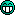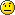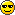## Portable XnView.db

Ask for help and post your question on how to use XnView Classic.

Moderators: XnTriq, helmut, xnview

markdfink
Posts: 9
Joined: Fri Mar 13, 2009 5:31 pm

### Portable XnView.db

I use an external hard drive with copies of all my images so that at lunch when I'm at my day job, I can look up and work on images. Then I synchronize the external drive with my internal drive at home. (I use Beyond Compare 2, which works great). What I noticed is that after launching XnView, I don't see the categories that I created while at work earlier today. Where is the category information stored? It doesn't seem to be in the XnView.db file.

My setup is that at home, the internal drive is H and the external drive is the G, but at work, it the external drive is H. So all paths in the XnView.db file point to H:\... Once I synchronize at home, I figured that XnView wouldn't notice any difference, since the "active" drive is always H.

Categories are what really got my interest in XnView, because Adobe Bridge doesn't have anything like it. Plus, Bridge is SO SLOW and a memory hog. XnView is the perfect!

Thanks,

MarkClo
XnThusiast
Posts: 4441
Joined: Sun Oct 17, 2004 4:57 am
Location: Bordeaux, France

### Re: Portable XnView.dbmarkdfinkHello !

• That's stored in a special file <category.db>, it might be located either in the XnView folder, or in the
<…\User_name…\XnView\> folder, according to your choices in the Options >> System Integration…KR
Claude
Clo
Old user ON SELECTIVE STRIKE till further notice
obelisk
Posts: 455
Joined: Fri Jan 09, 2009 9:54 am

### Re: Portable XnView.db

you can make it all in custom folder, to make a true portable xnview.
Zottt
Posts: 25
Joined: Tue Mar 17, 2009 8:15 pm

### Re: Portable XnView.db

Hi Obelisk,

XNView is close to portable as the folders are stored with driveletters and absolute paths in xnview.db and category.db.
I'd appreceate if this would be changed to relative paths like for example in the sld-files.

Zotttoops66
XnThusiast
Posts: 2005
Joined: Tue Jul 17, 2007 1:17 am
Location: France

### Re: Portable XnView.db

Zottt wrote:...XNView is close to portable as the folders are stored with driveletters and absolute paths in xnview.db and category.db.
I'd appreceate if this would be changed to relative paths like for example in the sld-files...Zottt
Hello,
+1, I agree
XnViewMP Linux X64 - Debian - X64
markdfink
Posts: 9
Joined: Fri Mar 13, 2009 5:31 pm

### Re: Portable XnView.db

Success! Thanks so much for pointing me in the right direction.

Mark
obelisk
Posts: 455
Joined: Fri Jan 09, 2009 9:54 am

### Re: Portable XnView.db

"changed to relative paths"
how would this work?
unless the xnview.db and category.db are in the same drive as your pics.
I know mine aren't!!
Zottt
Posts: 25
Joined: Tue Mar 17, 2009 8:15 pm

### Re: Portable XnView.db

Hi Obelisk!
If you have them all togehter on stick or CD then they are.
That is what is meant by "portable".
Regards!
Zottt
Posts: 25
Joined: Tue Mar 17, 2009 8:15 pm

### Re: Portable XnView.db

The database structure is pretty straight forward. I'd change the foldername by myself if somebody could tell me which is the base-directory to be used.
horusofoz
Posts: 1
Joined: Sun May 17, 2009 1:57 am

### Re: Portable XnView.db

With permission of the software author one could package XnView into a PortableApps installer. This would create an entirely portable version of XnView,XnTriq
Moderator & Librarian
Posts: 6190
Joined: Sun Sep 25, 2005 3:00 am
Location: Ref Desk

### Re: Portable XnView.db

horusofoz wrote:With permission of the software author one could package XnView into a PortableApps installer. This would create an entirely portable version of XnView,Install XnView on USB-stick?
Zottt
Posts: 25
Joined: Tue Mar 17, 2009 8:15 pm

### Re: Portable XnView.db

Pop!I'm still looking for a way to have a portable XnView so i can have it together with it's configs and my pictures together with comments and ratings on usb stick and it will work with any driveletter for example.

My most recent xnview.ini please find below.

I noticed "CustomIniPathFolder=." works pretty well as a relative reference to have category.db in the working directory.
Unfortunately this doesn't work with PathCache. It appears that "SavingMode=2" requires PathCache to be assigned an absolute path.
I'd like to use "SavingMode=2" together with "PathCache=." so that XnView.db will be used from the working directory the same way like category.db.

Folders in XnView.db are stored as absolute references. So all ratings get lost when the usb-stick is assigned a different driveletter.
I'd like to suggest an option like "-config_path = ..." to replace all absolute references. It could be set at startup and hence point XnView to the current location of config and media files. All non-absolute references would be relative to config_path then.
For backwards compatibility it would default to the users home.

Thanks.

Zottt

Code: Select all

``````[AutoCrop]
Tolerance=0

[Browser]
ActionConvert=0
ActionSlide=0
ArrangeBy=7
AutoCollapse=0
AutoPlay=0
BColorFilter01=jpeg, 237 237 202
BColorFilter02=gif, 220 235 220
BColorFilter03=png, 232 222 220
BColorFilter04=bmp, 227 227 255
BColorFilter05=tiff, 192 197 235
BColorFilter06=tga, 230 255 255
BColorFilter07=iff, 247 235 235
BColorFilter08=sgi, 247 237 242
BColorFilter09=pcx, 235 230 230
BColorFilter10=psd, 255 255 227
BColorFilter11=pcd, 235 215 235
BColorFilter12=psp, 237 208 163
BColorFilter13=pict, 202 202 255
BColorFilter14=xpm, 242 227 21
BColorFilter15=xbm, 216 216 216
BColorFilter16=pbm, 191 191 182
BColorFilter17=pgm, 207 207 197
BColorFilter18=ppm, 235 225 159
BColorFilter19=ras, 255 255 227
BColorFilter20=wmf, 187 187 187
BColorFilter21=emf, 212 212 212
BColorFilter22=ico, 217 247 227
BColorFilter23=cur, 217 247 227
BColorFilter24=ani, 217 247 227
BColorFilter25=fpx, 229 255 255
BColorFilter26=jp2, 237 237 202
BackListColor=16777215
BackgroundColor=16777215
BorderColor=0
BorderSize=0
CRAW=1
ClickRename=1
Custom0=<Focal Length>mm f/<F-Number> <Exposure Time>s <ISO Value>iso
Custom1=<Width>x<Height> - <Size KB>
Custom2=
Custom3=
Custom4=
EnableAutoRefresh=1
FilterBy=5
FitVideo=0
FitWindowOption=1
FlatStyle=0
FullPreview=0
FullRowSelect=1
Height=665
HidePreviewScrollBar=0
HighQuality=0
IconHeight=69
IconInfo=268435455
IconWidth=92
LabelsAlignment=1
LabelsSpacing=1
Layout=3
ListHeight=256
ListViewHeight=417
ListViewWidth=746
ListWidth=256
LockLayout=0
NavigateSubFolder=0
OldTreeTab=0
OldValue=0
OneClick=1
OpenAction0=0
OpenAction1=0
OpenAction2=0
OpenAction3=1
OpenAction4=0
OpenAction5=1
OpenAction6=0
PasteOnSubfolder=0
PreviewColor=8421504
PreviewHeight=215
PreviewWidth=0
PrintInfo=0
RecognizeByExt=1
RenameHideExtension=0
RotationKeepDate=1
RotationUseEXIF=0
RotationUseLossless=1
SharpenThumb=0
SharpenValue=50
ShowDesktop=0
ShowDirList=1
ShowFilename=1
ShowGridLines=1
ShowHexaView=0
ShowHiddenFile=0
ShowPreview=1
ShowSmallToolbar=1
ShowTabTree=1
ShowTabView=1
ShowToolTips=1
Spacing=4
StartupDirectory=
StartupIn=1
StatusInfo=0
SyncWithView=1
TabTreeLabel=0
TagSetting=1
TextBackColor=12632256
TextColor=0
Thumb41Folder=1
ThumbConfig=10 5 50
ThumbGamma=1.00
ThumbnailColor=16777215
TimerForPreview=200
TooltipText=<Filename With Ext>, <Size> Byte, <Modified Date>||<Format>||<Width>x<Height>, <BPP> Bit
TreeViewHeight=275
TreeViewWidth=200
TxtExt=txt c c++ cc h cpp pas js css php ini
UpdateTreeViewWhenRefresh=1
UseBackgroundColor=0
UseBytes=0
UseColor=1
UseFileDB=1
UseGammaOnThumb=0
UseInch=0
UseRating=1
UseRenameDlg=1
UseTag=0
UseThumbnailColor=0
VHidePanel=1
VListLayout=0
VideoFrame=0
VideoPlayBar=1
ViewColumn=255
Viewas=1
Width=960
X=0
Y=0
column0=0
column1=0
column10=0
column11=0
column2=0
column3=0
column4=0
column5=0
column6=0
column7=0
column8=0
column9=0
tcolumn0=0
tcolumn1=0
tcolumn2=0
tcolumn3=0

[Cache]
Compression=1
IsActive=0
MaxDir=99
SavingMode=2

[Canvas]
Alpha=255
KeepRatio=1
Position=4

[Capture]
Area=0
ClientArea=0
Delay=5
Directory=
FormatName=jpeg
HideCursor=1
HideXnView=1
HotKey=9
ImportFormat=1
IncludeCursor=0
Method=0
Multiple=0
Name=capture_[mdY_HMS]
SaveIntoFile=0

[ContactSheet]
BackgroundColor=16777215
Columns=4
Flags=4
FontBold=0
FontHeight=-13
FontItalic=0
FontName=Courier
FootNote=
Height=1000
MarginHeight=16
MarginWidth=16
Rows=4
SpacingHeight=8
SpacingWidth=8
Text=<Filename>
TextBackgroundColor=16777215
TextColor=0
ThumbnailBackgroundColor=12632256
Width=1000

[File]
ConfirmFileDelete=1
CopyCompanionFile=0
CopyTo=
DeleteRecycleBin=1
DirName_0=
DirName_1=
DirName_2=
DirName_3=
DirName_4=
DirName_5=
DirName_6=
DirName_7=
DirName_8=
DirName_9=
KeepDate=0
LosslessBak=1
MoveTo=
PasteNoCopy=0

[FileSelector]
IncludeSubDirectories=0

[Full]
BackColor=0
DualMStretch=0
DualMUse=1
Fit=0
HidePlayBar=0
HideScrollBar=1
SizeDelay=2048
UseDelay=1

[Fullscreen]
FontBold=0
FontCharSet=1
FontHeight=-13
FontItalic=0
FontName=Courier
IsTransparent=1
Opacity=255
Position=0
TextBackColor=0
TextColor=16777215

[Install]
ViewAsName=1

CRAWAutoBalance=0
CRAWBlueScaling=1.00
CRAWBrightness=0.80
CRAWCameraAutoBright=1
CRAWCameraBalance=1
CRAWGamma=0.60
CRAWHighResolution=1
CRAWRedScaling=1.00
ChannelOrder=0
ChannelType=0
DjVuMaxDpi=0
Dpx32Pack=0
EXIFDpi=0
EXIFRotate=1
ExrGamma=1.00
GrobBpp=0
HdrGamma=1.00
Height=256
ICCDef=0
ICCDefProfileName=
ICCFlags=0
ICCProfile=0
ICCProfileName=
IcoIn32bits=1
IgnoreError=0
Lut10to8Name=
Offset=0
PcdBase=2
PddPage=0
PngComposeWithAlpha=0
PngComposeWithTransparency=0
PostscriptDpi=72
PostscriptPageSize=0
Preview=1
RawBpp=24
UseEPSCIE=0
UseEmbeddedThumbnail=1
UseLut=0
UseOrgIfSmall=0
WMFHighResolution=0
Width=256
YuvHeight=576
YuvOffset=0
YuvWidth=720

[OpenWith]
OpenWith_0=

[Player]
SoundOn=1
Volume=100

[Process]
AutoView=0
Deinterlace=0
FilterAutoView=0
LastValue_0=-255
LastValue_1=-255
LastValue_10=-255
LastValue_11=-255
LastValue_12=-255
LastValue_13=-255
LastValue_14=-255
LastValue_15=-255
LastValue_16=-255
LastValue_17=-255
LastValue_18=-255
LastValue_19=-255
LastValue_2=-255
LastValue_20=-255
LastValue_21=-255
LastValue_22=-255
LastValue_23=-255
LastValue_24=-255
LastValue_25=-255
LastValue_26=-255
LastValue_27=-255
LastValue_28=-255
LastValue_29=-255
LastValue_3=-255
LastValue_30=-255
LastValue_31=-255
LastValue_32=-255
LastValue_33=-255
LastValue_34=-255
LastValue_35=-255
LastValue_36=-255
LastValue_37=-255
LastValue_38=-255
LastValue_39=-255
LastValue_4=-255
LastValue_40=-255
LastValue_41=-255
LastValue_42=-255
LastValue_43=-255
LastValue_44=-255
LastValue_5=-255
LastValue_6=-255
LastValue_7=-255
LastValue_8=-255
LastValue_9=-255
ShowPreview=1

[Resize]
DpiUnit=0
KeepDocSize=0
KeepRatio=1
PrintUnit=1
Resample=7
Unit=1
UseResample=1

[Save]
BmpCompress=0
ChannelOrder=0
ChannelType=0
DefaultFormat=jpeg
DpxIn10Bits=0
DpxMSBF=0
EcwTargetRatio=20
FpxQuality=100
GifInterlaced=0
GifUseTransparentIndex=0
IffCompress=0
IwcQuality=100
Jpeg2000FileSize=100
Jpeg2000Mode=1
Jpeg2000Quality=80
Jpeg2000Ratio=10
JpegDCTMethod=0
JpegKeepEXIF=1
JpegKeepICC=1
JpegKeepIPTC=1
JpegKeepXMP=0
JpegProgressive=0
JpegQuality=80
JpegRebuildThumb=1
JpegSmoothFactor=0
JpegSubSampling=0
JpegXRColor=0
JpegXRFilter=1
JpegXRQuality=100
KeepOrgDate=0
LdfQuality=1
LdfjpmBackFactors=1
LdfjpmBitonalCoder=2
LdfjpmForeFactors=1
LdfjpmQuality=8
Lut8to10Name=
LwfCompression=0
LwfJp2Compression=0
LwfJp2Rate=10
LwfJp2Size=4096
LwfMode=0
LwfQuality=70
LwfRate=10
LwfSize=1024
Offset=0
OptimizeHuffmanTable=0
PcxCompress=0
PdfCompress=4
PdfJpegQuality=80
PngCompressionLevel=6
PngInterlaced=0
PngTransparentIndex=0
PngUseTransparentIndex=0
PpmAscii=0
RemoveAlpha=0
RgbCompress=1
SoftCompress=1
TgaCompress=0
TgaOrigin=0
Tiff1Strip=0
TiffBinaryCompress=0
TiffCompress=0
TiffJpegQuality=80
UseLut=0
UseOrgJPEGQuality=0
WMPQuality=100
WebPQuality=100
WicQuality=100
WlmFactor=1

[Scr]
PlayMode=0
WindowHeight=480
WindowWidth=640

[Slide]
AudioLoop=0
BackgroundColor=0
Center=1
EffectFlag=1
FitSmall=0
FitSmallVideo=0
FontBold=0
FontCharSet=1
FontHeight=-13
FontItalic=0
FontName=Courier
Frame=1
Full=1
HighQuality=1
IsTransparent=1
Loop=1
MouseNav=0
NoError=0
NoTitle=1
OnTop=0
Opacity=255
PlayMode=0
Position=0
Random=0
SLDRelativePathname=0
ShowText=1
SortOrder=0
SoundFilename=
Text=<Directory><Filename>
TextBackColor=0
TextColor=16777215
Timer=5.00
UseGammaInEXE=1
UseSound=0
UseTimer=1
Watch=0
WinHeight=480
WinWidth=640

[Start]
AllFormats=0
AlwaysOnTop=0
BStatusBar=1
BToolBar=1
BarFilename=
CColor-0=0
CColor-1=0
CColor-10=0
CColor-11=0
CColor-12=0
CColor-13=0
CColor-14=0
CColor-15=0
CColor-2=0
CColor-3=0
CColor-4=0
CColor-5=0
CColor-6=0
CColor-7=0
CColor-8=0
CColor-9=0
CheckUpdate=0
Custom1Extensions=HTM HTML MHT MHTML XML XHTML EML NWS URL
Custom2Extensions=
CustomIniPathFolder=.
DirName_0=
DirName_1=
DirName_2=
DirName_3=
DirName_4=
DirName_5=
DirName_6=
DirName_7=
DirName_8=
DirName_9=
ENTER=1
FileName_0=
FileName_1=
FileName_2=
FileName_3=
FileName_4=
FileName_5=
FileName_6=
FileName_7=
FileName_8=
FileName_9=
Filter0=639
Filter1=895
Filter2=589
Filter3=287
FlatButtonInToolbar=1
Height=768
InFullscreen=0
LMB=1
Language=de
LastCheck=0
LaunchBrowserAtStartup=1
MMB=0
MaxFilesize0=99999999
MaxFilesize1=99999999
Maxi=0
MaximizeXnviewAtStartup=0
Only1ESC=1
OnlyOneInstance=0
OpenAll=1
ParamsSavingMode=2
PathAvi=
PathBrowse=
PathCache=.
PathConvertSave=
PathPalette=
PathSave=
PathSaveAll=
PathScript=
PathSlide=
PathSlideScript=
PurgeMostRecentlyUsed=0
SaveAsUseNewName=1
SavePosition=0
SavedFileType=:gif:jpeg:png:tiff:bmp:emf:pcx:
SavingMode=1
SelectedShowAgain=0
ShowAgain=268435455
ShowSaveDlg=0
ShowTextInToolbar=0
ShowToolTipsInToolbar=1
TabBar=1
TabBarCfg=1
TabBarDefault=0
TabBarMaxChar=32
TagShowFlags=0
ToolbarBrowserConfig=-536870913
ToolbarBrowserConfigHigh=-1
ToolbarName=
ToolbarViewConfig=-369098769
ToolbarViewConfigHigh=-1
UseDifferentPosition=0
UseMostRecentlyUsedFiles=0
UseNewMRUF=1
UseSameAsOrg=0
UseSavedPath=0
VStatusBar=1
VToolBar=1
Version=199
ViewHeight=768
ViewMaxi=0
ViewWidth=960
ViewX=-1
ViewY=-1
Width=960
X=-1
Y=-1

[View]
AppFitToImage=0
BackColor=0
BackgroundColor=8421504
CRAW=2
CacheBehind=0
ColorInfo=0
CopyClipboard=0
DeleteGoNext=1
DirKeyFlag=1
DirKeyVFlag=2
DisableGifAnimation=0
EnableBmpAnimation=0
Fit=3
Gamma=1.00
GridColor=255
GridSize=2
GridSpacingX=80
GridSpacingY=80
GridType=1
HideScrollBar=0
HighZoomQuality=1
IgnoreAlpha=0
ImageLoop=0
KeepGridForNextPrevious=0
LeftButtonFlag=1
LockZoom=0
MultipageView=0
NoMultipleView=0
OneViewMultiple=0
OnlyOneView=0
OpenHexaViewIfNotPicture=0
PlayMovie=1
PlaySound=1
ResetXY=0
RightButtonFlag=0
ScrollVal=10
ShowColorInfo=0
ShowText=1
SlideDelay=1000
SlideDirection=0
SlideLoop=1
SpaceKeyFlag=0
StatusInfo=0
TTitle=<Filename With Ext>
Text=<Directory><Filename>||Tag: <Tag status>
UnitsSize=0
UseAlpha=1
UseBgImg=0
UseDPIRatio=1
UseGamma=0
UseTranspIndex=1
UseUndo=1
UseZoomStep=0
UserSelHeight=0
UserSelRatioX=0
UserSelRatioY=0
UserSelWidth=0
UserSelXdpi=0
UserSelYdpi=0
VideoFitAll=0
VideoLoop=0
VideoPlayBar=1
WheelMouseFlag=2
ZoomFStep=10
ZoomRStep=1.41
``````